# Confidence Intervals Explained: Examples & Interpretation

The confidence interval (CI) is a range of values that’s likely to include a population value with a certain degree of confidence. It is often expressed as a % whereby a population mean lies between an upper and lower interval.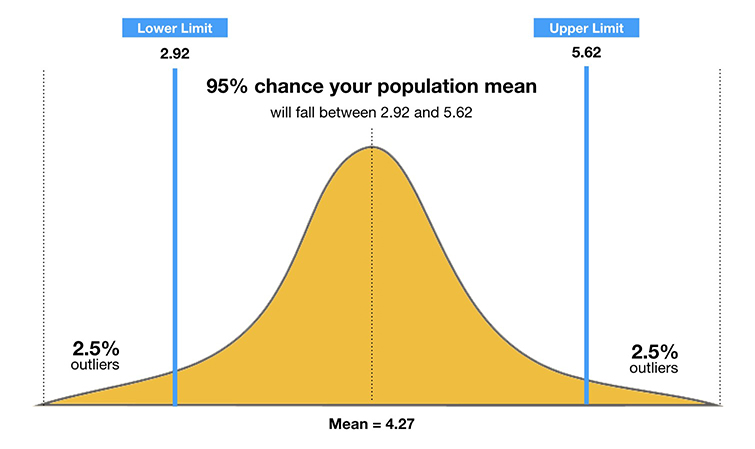## What is a 95% confidence interval?

The 95% confidence interval is a range of values that you can be 95% confident contains the true mean of the population. Due to natural sampling variability, the sample mean (center of the CI) will vary from sample to sample.

The confidence is in the method, not in a particular CI. If we repeated the sampling method many times, approximately 95% of the intervals constructed would capture the true population mean.

Therefore, as the sample size increases, the range of interval values will narrow, meaning that you know that mean with much more accuracy than a smaller sample.

We can visualize this using a normal distribution (see the below graph).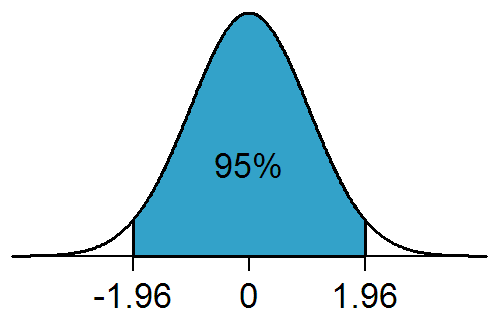For example, the probability of the population mean value being between -1.96 and +1.96 standard deviations (z-scores) from the sample mean is 95%.

Accordingly, there is a 5% chance that the population mean lies outside of the upper and lower confidence interval (as illustrated by the 2.5% of outliers on either side of the 1.96 z-scores).

## Why use confidence intervals?

It is more or less impossible to study every single person in a population, so researchers select a sample or sub-group of the population.

This means that the researcher can only estimate a population’s parameters (i.e., characteristics), the estimated range being calculated from a given set of sample data.

Therefore, a confidence interval is simply a way to measure how well your sample represents the population you are studying.

The probability that the confidence interval includes the true mean value within a population is called the confidence level of the CI.

You can calculate a CI for any confidence level you like, but the most commonly used value is 95%. A 95% confidence interval is a range of values (upper and lower) that you can be 95% certain contains the true mean of the population.

## How to calculate

To calculate the confidence interval, start by computing the mean and standard error of the sample.

Remember, you must calculate an upper and low score for the confidence interval using the z-score for the chosen confidence level (see table below).

Confidence Level Z-Score
0.90 1.645
0.95 1.96
0.99 2.58

## Confidence Interval Formula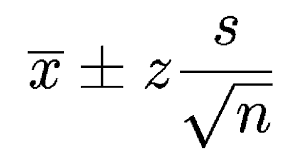Where:

• X is the mean
• Z is the chosen Z-value (1.96 for 95%)
• s is the standard error
• n is the sample size

For the lower interval score, divide the standard error by the square root on n, and then multiply the sum of this calculation by the z-score (1.96 for 95%). Finally, subtract the value of this calculation from the sample mean.

### An Example:

• X (mean) = 86
• Z = 1.960 (from the table above for 95%)
• s (standard error) = 6.2
• n (sample size) = 46

Lower Value: 86 – 1.960 × 6.2 √46 = 86 – 1.79 = 84.21

Upper Value: 86 + 1.960 × 6.2 √46 = 86 + 1.79 = 87.79

So the population mean is likely to be between 84.21 and 87.79

## Population mean and sample mean

How can we be sure that the population mean is similar to the sample mean?

The narrower the interval (upper and lower values), the more precise our estimate is.

As a general rule, as the sample size increases, the confidence interval should become more narrow.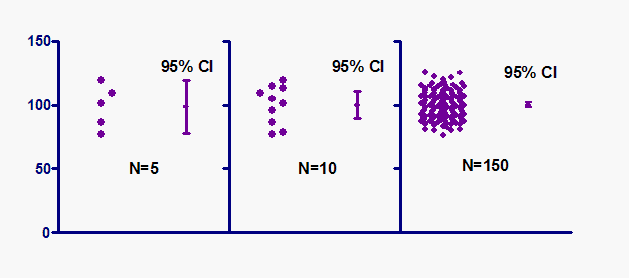Therefore, with large samples, you can estimate the population mean more precisely than with smaller samples. Hence, the confidence interval is quite narrow when computed from a large sample.

## How to report

The APA 6 style manual states (p.117):

“ When reporting confidence intervals, use the format 95% CI [LL, UL] where LL is the lower limit of the confidence interval and UL is the upper limit.”

For example, one might report a 95% CI [5.62, 8.31].

Confidence intervals can also be reported in a table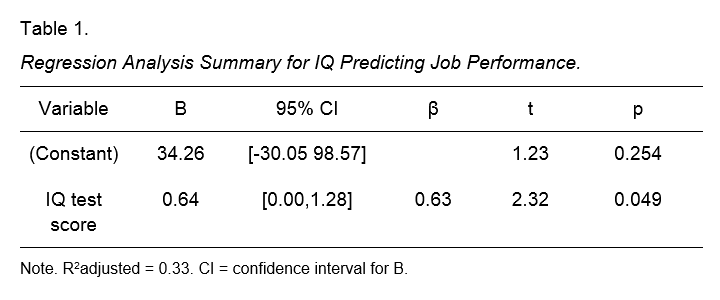## Further Information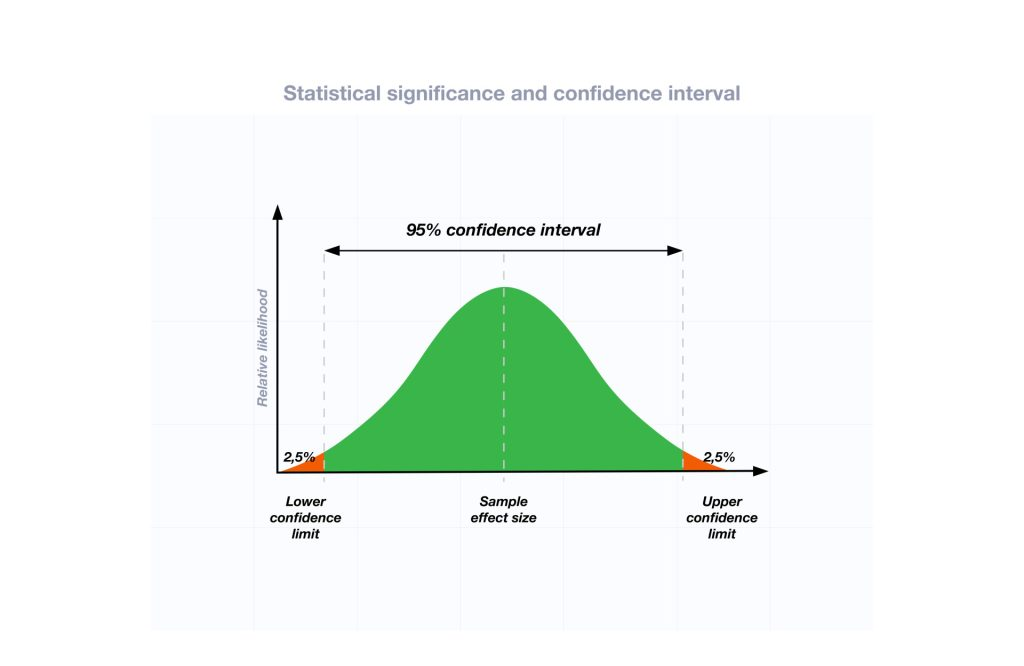Saul Mcleod, PhD

BSc (Hons) Psychology, MRes, PhD, University of Manchester

Educator, Researcher

Saul Mcleod, Ph.D., is a qualified psychology teacher with over 18 years experience of working in further and higher education.Saul Mcleod, PhD

Educator, Researcher

BSc (Hons) Psychology, MRes, PhD, University of Manchester

Saul Mcleod, Ph.D., is a qualified psychology teacher with over 18 years experience of working in further and higher education.